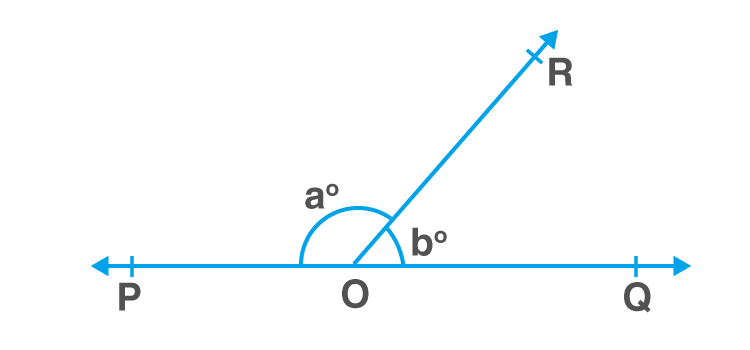# Important 2 Marks Questions for CBSE 9 Maths

Central Board of Secondary Education (CBSE) is responsible for conducting an examination for the schools affiliated to the central board. It is of prime importance for the students to excel in the field of education for their brightening future. Maths requires proper understanding and skills rather than just memorizing like other subjects. Practising important questions would be an excellent support for the students and boost up their confidence. We at BYJU’S provide students of class 9th with 2 marks important questions to practice. Practising them will be helpful for students to excel in their examination. Students preparing for CBSE Class 9 Maths Board Examination are advised to practice the question given below:

Question 1- Express the rational number $$0.\bar{45}$$ in the form $$\frac{p}{q}$$, where p and q are natural number.

Question 2- Find the remainder, when the polynomial $$2x^{4}+ x^{3} + 4x^{2}- 3x -2$$ is divided by $$x-3$$ (without applying long division).

Question 3- Find the value of k if (x-1) is a factor of $$4x^{3} + 3x^{2}- 4x + k$$.

Question 4- In which quadrant or on which axis each of the given points lies?

(i) (-2,4)

(ii) (-8,0)

(iii) (1,-7)

(iv) (-7,-2)

Question 5- If a point C lies between two points A and B such that AC=BC, then prove that AC = 1/2 AB.

Question 6- If $$x^{\frac{a}{b}} = 1$$, and $$b \neq 0$$, then find the value  of ‘a’.

Question 7- If the opposite angles of a parallelogram are $$(63 – 3x)^{\circ}$$ and $$(4x – 7)^{\circ}$$. Find all the angles of the parallelogram.

Question 8- Construct a triangle in which the three sides are of length 6 cm, 4 cm and 2.8 cm.

Question 9- Represent $$\sqrt{5}$$ on a number line.

Question 10- The points scored by a Kabaddi team in a series of matches are as follows

17, 27, 7, 27, 15, 5, 14, 8, 10, 24, 48, 10, 8, 7, 18, 28

Find the median and mode of the data.

Question 11- Find the value of ‘a’ such that x = 1  and y = 1 is a solution of the linear equation

9ax + 12ay = 63

Question 12- Evaluate $$(104)^{3}$$ using suitable identity.

Question 13- If the angles of a triangle are in the ratio 2 : 3 : 4, then find the angles of the triangle.

Question 14- In the given figure, if $$\angle POR$$ and $$\angle QOR$$ form pair and $$a – b = 80^{\circ}$$, then find the value of a and b.Question 15- Expand $$\left ( \frac{1}{x} + \frac{y}{3} \right )^{3}$$

Question 16- Find the two irrational number between 0.5 and 0.55.

1. Arob Mohanty

Very good

2. Ojaswani sharma

I find this very useful and questions were interesting

Thankyou

4. Kanaparthi Abhiram

They are really useful for me

5. shivansh agrahari

excellent sir amazing

Wow it is help in my study

7. Gouri Vinu

Thanks for helping me out

8. Plz give solutions too so that we can check our ans and see the ques that we are unable to do

9. Dhanushree m

It was really useful, interesting questions Thank you

10. Very useful for exams. thank uhhhh

11. Anish Ghosh

Very helpful questions for my exam. Thanks.